# RS Aggarwal Class 6 Solutions Angles And Their Measurement

## RS Aggarwal Class 6 Solutions Chapter 13

In this chapter, the students will learn about different types of angles like an acute angle, right angle and obtuse angle. Learn the concepts of angles and their measurement by solving the questions from RS Aggarwal class 6. Solving the RS Aggarwal solutions will also help the students to practice questions of various difficulty level. To help students solve all the questions and to score good marks we have provided the RS Aggarwal class 6 solutions chapter 13 Angles and their Measurements.

## EXERCISE – 13 A

QUESTION – 1:

Name three examples of angles from your daily life.

Solution:

(1) Angle formed at the vertex of our elbow with the upper arm and the lower arm as the two rays. This angle will vary as per the position of our arm.

(2) Angle formed between the two hands of the clock that are hinged at a point.

(3) Angle formed between the two hands of a windmill. They are also hinged at a point, which is called the vertex of that angle.

QUESTION – 2: Name the vertex and the arms of $angle ABC$, given in the figure below.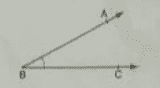Solution:

The vertex is B.

Arms of $angle ABC$ are rays $vec{BA}:and:vec{BC}$.

QUESTION – 3: How many angles are formed in each of the figures given below? Name them.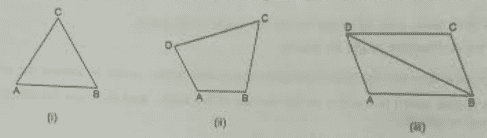Solution:

(i) Here, three angles are formed. They are $angle ABC,angle ACB:and:angle BAC$

(ii) Here, four angles are formed. They are $angle ABC,angle BCD,angle CDA:and:angle DAB$

(iii) Here, eight angles are formed. They are $angle ABC,angle BCD,angle CDA,angle DAB,angle ABD,angle ADB,angle CDB,angle CBD$

QUESTION – 4: In the given figure, list the points which

(i) are in the interior of $angle AOB$

(ii) are in the exterior of $angle AOB$

(iii) lie on $angle AOB$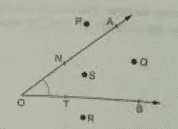Solution:

(i) Q and S are in the interior of $angle AOB$

(ii) P and R are the exterior of $angle AOB$.

(iii) A, O, B, N and T lie on the angle $angle AOB$.

QUESTION – 5: See the adjacent figure and state which of the following statements are true and which are false.

(i) Point C is in the interior of $angle AOC$

(ii) Point C is in the interior of $angle AOD$

(iii) Point D is in the interior of $angle AOC$

(iv) Point B is in the exterior of $angle AOD$

(v) Point C lies on $angle AOB$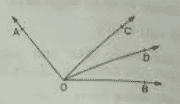Solution:

(i) False

Point C is on the angle $angle AOC$.

(ii) True

Point C lies in the interior of $angle AOD$.

(iii) False

Point D lies in the exterior of $angle AOC$.

(iv) True

Point B lies in the exterior of $angle AOD$.

(v) False

Point C lies in the interior of $angle AOB$.

QUESTION – 6: In the adjoining figure, write another name for:

(i) $angle 1$ (ii) $angle 2$ (iii) $angle 3$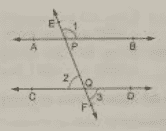Solution:

(i) $angle EPB$.

(ii) $angle PQC$.

(iii) $angle FQD$.

## EXERCISE – 13 B

QUESTION – 1: State the type of each of the following angles: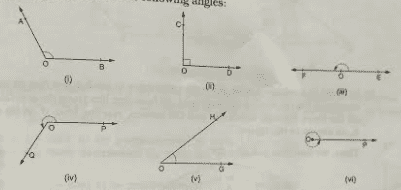Solution:

(i) $angle AOB$ is an obtuse angle since its measure is more than $90^{circ}$.

(ii) $angle COD$ is a right angle since its measure is $90^{circ}$.

(iii) $angle FOE$ is a straight angle since its measure is $180^{circ}$.

(iv) $angle POQ$ is a reflex angle since its measure is more than $180^{circ}$ but less than $360^{circ}$.

(v) $angle HOG$ is an acute angle since its measure is more than 0 but less than $90^{circ}$.

(vi) $angle POP$ is a complete angle since its measure is $360^{circ}$.

QUESTION – 2: Classify the angles whose magnitudes are given below:

(i) $30^{circ}$ (ii) $91^{circ}$ (iii) $179^{circ}$

(iv) $90^{circ}$ (v) $181^{circ}$ (vi) $360^{circ}$

(vii) $128^{circ}$ (viii) $left ( 90.5 right )^{circ}$

(ix) $left ( 38.3 right )^{circ}$

(x) $80^{circ}$ (xi) $0^{circ}$

(xii) $15^{circ}$

Solution:

(i) Acute angle

This is because its measures is less than $90^{circ}$ but more than $0^{circ}$.

(ii) Obtuse angle

This because its measure is more than $90^{circ}$ but less than $180^{circ}$.

(iii) Obtuse angle

This because its measure is more than $90^{circ}$ but less than $180^{circ}$.

(iv) Right angle

This is because its measure is $90^{circ}$.

(v) Reflex angle

This because its measure is more than $180^{circ}$ but less than $360^{circ}$.

(vi) Complete angle

This is because its measure is $360^{circ}$.

(vii) Obtuse angle

This because its measure is more than $90^{circ}$ but less than $180^{circ}$.

(viii) Obtuse angle

This because its measure is more than $90^{circ}$ but less than $180^{circ}$.

(ix) Acute angle

This is because its measures is less than $90^{circ}$ but more than $0^{circ}$.

(x) Acute angle

This is because its measures is less than $90^{circ}$ but more than $0^{circ}$.

(xi) Zero angle

This is because its measure is zero.

(xii) Acute angle

This is because its measures is less than $90^{circ}$ but more than $0^{circ}$.

QUESTION – 3: How many degrees are there in

(i) one right angle? (ii) two right angles?

(iii) three right angles? (iv) four right angles?

(v) 2/3 right angles? (vi) $1frac{1}{2}$ right angles?

Solution:

(i) One right angle has $90^{circ}$.

(ii) Two right angles have $90^{circ}+90^{circ}=180^{circ}$.

(iii) Three right angles have $90^{circ}+90^{circ}+90^{circ}=270^{circ}$

(iv) Four right angles have $90^{circ}+90^{circ}+90^{circ}+90^{circ}=360^{circ}$

(v) $frac{2}{3}times 90=60^{circ}$

(vi) $left (1frac{1}{2}right )right:angles=frac{3}{2}times 90=135^{circ}$

QUESTION – 4: How many degrees are there in the angle between the hour hand and the minute hand of a clock, when it is

(i) 3 o’clock (ii) 6 o’clock (iii) 12 o’clock (iv) 9 o’clock

Solution:

(i) At 3 o’clock the angle formed between the hour hand and the minute hand is right angle, i.e. $90^{circ}$.

(ii) At 6 o’clock the angle formed between the hour hand and minute hand is a straight line, i.2. $180^{circ}$.

(iii) At 12 o‘clock the angle formed between the hour hand and minute hand is a complete angle, i.2. $0^{circ}$.

This is because the hour hand and the minute hand coincides to each other at 12 o’clock.

(iv) At 9 o’clock the angle formed between the hour hand and the minute hand is right angle, i.e. $90^{circ}$.

QUESTION – 5: Using only a ruler, draw an acute angle, an obtuse angle and a straight angle.

Solution:

(i) Acute angle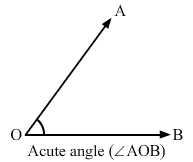(ii) Obtuse angle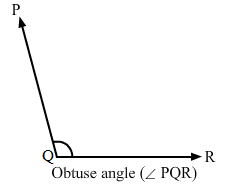(iii) Straight angle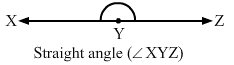## EXERCISE – 13 C

QUESTION – 1: Measure each of the following angles with the help of a protractor and write the measure in degrees: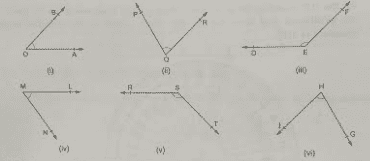Solution:

(i) $angle AOB=45^{circ}$

(ii) $angle PQR=75^{circ}$

(iii) $angle DEF=135^{circ}$

(iv) $angle LMN=55^{circ}$

(v) $angle TSR=135^{circ}$

(vi) $angle GHI=75^{circ}$

We have measured all the above by placing the protractor on one of the arms of the angle and measuring the angle through the other arm that coincides with the angle on the protractor.

QUESTION – 2: Construct each of the following angles with the help of a protractor:

(i) $25^{circ}$ (ii) $72^{circ}$ (iii) $90^{circ}$

(iv) $117^{circ}$ (v) $165^{circ}$ (vi) $23^{circ}$

(vii) $180^{circ}$ (viii) $48^{circ}$

Solution:

Steps to follow:

Draw a ray QP with Q as the initial point.

Place the protractor on QP. With its centre on Q, mark a point R against the given angle mark of the protractor.

Join RQ. Now, PQR is the required angle.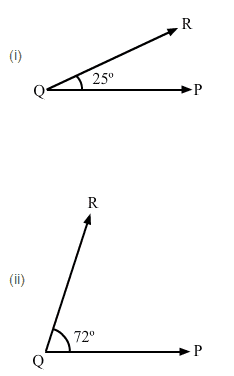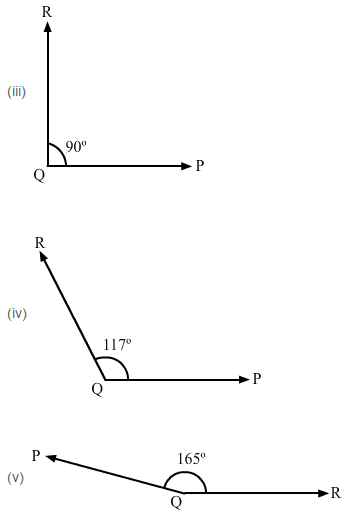QUESTION – 3: Measure $angle ABC$ given in the adjoining figure and construct an angle DEF equal to $angle ABC$.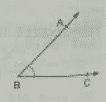Solution:

We can see that $angle ABC=47^{circ}$.

Steps to follow to construct angle $angle DEF$ equal to $angle ABC$:

Draw a ray EF with E as its initial point.

Place the protractor on EF. With the centre at E, mark a point D against the angle $47^{circ}$ of the protractor.

Join DE. $angle DEF=47^{circ}=angle ABC$ is the required angle.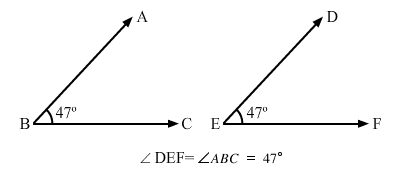QUESTION – 4: Draw a line segment AB = 6cm. Take a point C on AB such that AC = 4cm. From C, draw $CDperp AB$

Solution:

Draw a line segment AB of length 6 cm.

Mark pint C on AB such that AC is equal to 4 cm.

Place the protractor an AB such that the centre of the protractor is on C and its base lies along AB.

Holding the protractor, mark a point D on the paper against the $90^{circ}$ mark of the protractor.

Remove the protractor and draw a ray CD with C as the initial point.

Now, $CDperp AB$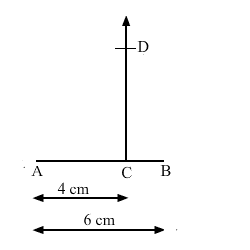## EXERCISE – 13 D

OBJECTIVE QUESTIONS

MARK () AGAINST THE CORRECT ANSWER IN EACH OF THE FOLLOWING:

QUESTION – 1: Where does the vertex of an angle lie?

(a) In its interior (b) In its exterior (c) On the angle (d) None of these

Solution:

(c) On the angle

Vertex is the initial point of two rays between which the angle formed. Therefore, it lies on the angle.

QUESTION – 2: The figure formed by two rays with the same initial point is called

(a) a ray (b) a line (c) an angle (d) none of these

Solution:

(c) An angle

The initial point is called the vertex.

QUESTION – 3: An angle measuring $180^{circ}$ is called

(a) a complete angle (b) a reflex angle (c) a straight angle (d) none of these

Solution:

(c) Straight angle

QUESTION – 4: An angle measuring $90^{circ}$ is

(a) a straight angle (b) a right angle (c) a complete angle (d) a reflex angle

Solution:

(b) Right angle

QUESTION – 5: An angle measuring $91^{circ}$ is

(a) an acute angle (b) an obtuse angle (c) a reflex angle (d) none of these

Solution:

(b) An obtuse angle

This is because it is more than $90^{circ}$ but less than $180^{circ}$.

QUESTION – 6: An angle measuring $270^{circ}$ is

(a) an obtuse angle (b) an acute angle (c) a straight angle (d) a reflex angle

Solution:

(d) A reflex angle

This is because it is more than $180^{circ}$ but less than $360^{circ}$.

QUESTION – 7: The measure of a straight angle is

(a) $90^{circ}$ (b) $150^{circ}$ (c) $180^{circ}$

(d) $360^{circ}$

Solution:

(c) $180^{circ}$

QUESTION – 8: An angle measuring $200^{circ}$ is

(a) an obtuse angle (b) an acute angle (c) a reflex angle (d) none of these

Solution:

(c) A reflex angle

This is because it is more than $180^{circ}$ but less than $360^{circ}$.

QUESTION – 9: An angle measuring $360^{circ}$ is

(a) a reflex angle (b) an obtuse angle (c) a straight angle (d) a complete angle

Solution:

(d) A complete angle

This is because it completes the rotation of $360^{circ}$.

QUESTION – 10: A reflex angle measure

(a) more than $180^{circ}$ but less than $270^{circ}$

(b) more than $180^{circ}$ but less than $360^{circ}$

(c) more than $90^{circ}$ but less than $180^{circ}$

(d) none of these

Solution:

(b) more than $180^{circ}$ but less than $360^{circ}$

QUESTION – 11: 2 right angles =?

(a) $90^{circ}$ (b) $180^{circ}$

(c) $270^{circ}$ (d) $360^{circ}$

Solution:

(b) 2 right angles = $2times 90^{circ}=180^{circ}left ( straight :line right )$

QUESTION – 12: 3/2 right angles =?

(a) $115^{circ}$ (b) $135^{circ}$

(c) $270^{circ}$ (d) $230^{circ}$

Solution:

(b) $135^{circ}$

$frac{3}{2}:right:angle=frac{3}{2}times 90^{circ}$

= $135^{circ}$

QUESTION – 13: If there are 36 spokes in a bicycle wheel, then the angle between a pair of adjacent spokes is

(a) $15^{circ}$ (b) $12^{circ}$

(c) $10^{circ}$ (d) $18^{circ}$

Solution:

(c) $10^{circ}$

Number of spokes = 36

Measure of the angle of the wheel = Complete angle = $360^{circ}$

Angle between a pair of adjacent spokes = Measure of angle / Number of spokes = $360^{circ}$ / 36 = $10^{circ}$

#### Practise This Question

Which of the following sequences is correct in making fabrics?